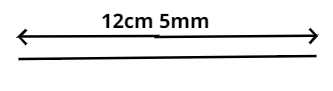QuestionAnswers

# Construct line segments whose lengths are: 12 cm 5 mmVerified
152.1k+ views
Hint : Use compass to do the construction.

Draw a line l on the paper and mark a point A on it.

Take a compass and place its metal point at zero mark of the ruler.

Adjust the compass such that the pencil point gets placed at the point which is five small points from the mark of 12 cm to 13 cm of the ruler.

Now, take the compass to l such that its metal point is on A.

Mark a small mark at B on l corresponding to the pencil point of the compass.

AB is the required line segment of 12 cm 5 mm.Note :Whenever we are asked to draw a line segment we have to take that measurement on the compass with the help of scale then put the needle of compass at starting point and next end on the end point mark the arc then join the point with arc with the help of scale you will get the required line. Actually it is the radius of the circle of which that arc is the part.